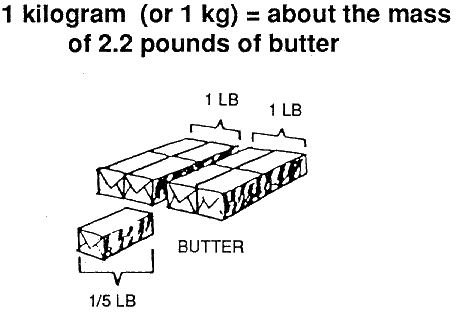# Convert Pounds to Kilograms and Kilograms to Pounds

## Teachers Love Unit Conversion Problems

Being able to change kilograms to pounds and pounds to kilograms will help you in school and in any future career in science. Here’s how to do it.

## A Coarse Approximation

A very easy-to-remember (and fairly accurate) approximation to remember is weight in pounds is twice the weight in kilograms.

So, roughly, 200 pounds is 100 kilograms, 2,000 pounds is a ton (a thousand kilos) and 50 kilograms is 100 pounds. This approximation of unit conversion is only good, however, when you need a general idea of someone’s or something’s weight or mass.

## The Definition

Introduced in 1959 in the USA and in 1963 in the UK, it is stated that one pound ”shall be 0.45359237 kilogram, exactly”. This means one kilogram is approximately 2.20462262 pounds.1 kg is equal to about 2.2 lbs.
•  1kg = 2.2lb
• 1lb = 0.45kg
• 1lb = 0.453 kg

Note that these are approximations as in reality, the pound is slightly more than 0.45kg, and a kilogram is slightly more than 2.2 pounds.

## Sample Problem:

Here’s a sample question you might get asked on a test:

John, living in Toronto, comes to visit his friend Jane in Buffalo. John knows his weight is 84 kilograms. Jane had read research stating that for her height of 5 feet, a healthy weight would be 1.5 times lower than John’s. How many pounds does Jane need to lose if her current weight is 139 pounds?

We will now solve the question in two ways, illustrating how to convert kilograms to pounds and then how to convert pounds to kilograms.

## Change Kilograms to Pounds

Let’s convert John’s weight into pounds. If one kilogram is 2.2 pounds, then 84 kilograms is 84 x 2.2 = 184.8 pounds.

Now, let’s divide it by 1.5, getting 123.2.

The next step is easy: Jane needs to lose 139-123.2 = 15.8 pounds.

## Change Pounds to Kilograms

Now, let’s solve the question in metric units:

John’s weight is 84 kg, so Jane wants to reach 84/1.5 = 56 kg.

Her current weight is 139 pounds, which is 139 x 0.45 = 62.6 kg. This means that she needs to lose 62.6-56 = 6.6 kilograms.

Converting back to pounds we get 6.6 x 2.2 = 14.52 pounds.

Okay, but wait a second…. Why is the answer different?

## The Drawbacks of Approximation

The answers differ because of approximation.

Note that 2.2 x 0.45 is 0.99, which gives us 1 percent of error.

The difference of over 10% between the answers is a great illustration for the need for caution when using approximation in converting pounds to kilograms.

## An Exact Solution

If we want to solve the above question with extreme accuracy, we should use more decimal places in our calculations. Let’s do it quickly:

84 / 0.45359237 = 185.1883002352972

185.1883002352972 / 1.5 = 123.4588668235314

139 – 123.4588668235314 = 15.54113317646855

Now go and tell any “Jane” you know that she needs to lose 15.54113317646855 pounds and see the reaction… if you dare!

## References and Image Credits

WordIQ.com, at https://www.wordiq.com/definition/Pound

Image Credits:

https://js082.k12.sd.us/Notes_and_Wrkshts/Metric_Factor-Lable/metric_system_info.htm

https://www.matthewb.id.au/converter/grams-to-pounds-and-ounces-converter.html